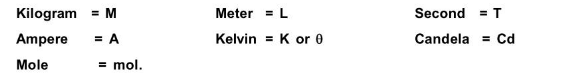Most Affordable JEE | NEET | 8,9,10 Preparation by Kota's Top IITian Doctor Faculties

# What are Dimensions of Physical Quantities ?## Dimensions of Physical Quantities

The limit of a derived quantity in terms of necessary basic units is called dimensional formula and the raised powers on the basic units are dimensions.

The basic units are represented as :Note :

1. A physical quantity may have a number of units but their dimensions would be same,

Ex. The units of velocity are: $\mathrm{cms}^{-1}, \mathrm{~ms}^{-1}, \mathrm{~km} \mathrm{~s}^{-1}$. But the dimensional formula is $\mathrm{M}^{0} \mathrm{~L}^{1} \mathrm{~T}^{1}$.

2. Dimension does not depend on the unit of quantity.